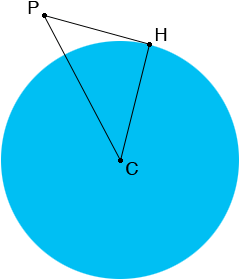SEARCH HOMEMath Central Quandaries & QueriesQuestion from Ali, a student: A plane is 10km above the earths surface, and you want to know how far away the horizon is, you know that the radius of the earth is about 6400km, how far away is the horizon along the earth's curved surface. My teacher has drawn a diagram; a circle representing the earth with one vertex at the center of the earth, another vertex outside the circle representing the plane (the distance between the earth and plane is 10km) and the last one is on the circle representing the horizon. Could you please explain how I would solve this problem, thanks!Hi Ali,

In my diagram C is the centre of the earth, P is the plane and H is the horizon as seen from the plane.|CH| = 6400 km, |PC| = 6400 + 10 km and since the line PH is tangent to the circle the angle CHP is a right angle. Use Pythagoras theorem to find the length of PH.

PennyMath Central is supported by the University of Regina and The Pacific Institute for the Mathematical Sciences.